NEET  >  Reflection & Refraction of Waves

# Reflection & Refraction of Waves - Notes | Study Physics Class 11 - NEET

 Table of contentsReflection and RefractionExtra Portion for IIT-Mains1 Crore+ students have signed up on EduRev. Have you?

Reflection and Refraction

• We can use a modified form of Huygens' construction to understand reflection and refraction of light. Figure (a) shows an incident wavefront which makes an angle 'i' with the surface separating two media, for example, air and water.
• The phase speeds in the two media are v1 and v2. We can see that when the point A on the incident wavefront strikes the surface, the point B still has to travel a distance BC = AC sin i, and this takes a time t = BC/v1 = AC (sin i)/v1. After a time t, a secondary wavefront of radius v2t with A as centre would have travelled into medium 2.
• The secondary wavefront with C as centre would have just started, i.e. would have zero radius. We also show a secondary wavelet originating from a point D in between A and C. Its radius is less than v2t. The wavefront in medium 2 is thus a line passing through C and tangent to the circle centred on A.
• We can see that the angle r¢ made by this refracted wavefront with the surface is given by AE = v2t = AC sin r¢. Hence, t =AC (sin r¢)/v2. Equating the two expressions for 't' gives us the law of refraction in the form sin i/ sir r¢ = v1/v2. A similar picture is drawn in shown figure (b) for the reflected wave which travels back into medium 1. In this case, we denote the angle made by the reflected wavefront with the surface by r, and we find that i = r. Notice that for both reflection and refraction, we see secondary wavelets starting at different times. Compare this with the earlier application (shown figure) where we start them at the same time.
• The preceding argument gives a good physical picture of how the refracted and reflected waves are built up from secondary wavelets.
• We can also understand the laws of reflection and refraction using the concept that the time taken by light to travel along different rays from one wavefront to another must be the same. (Fig) Shows the incident and reflected wavefronts when a parallel beam of light falls on a plane surface.
• One ray POQ is shown normal to both the reflected and incident wavefronts. The angle of incidence i and the angle of reflection r are defined as the angles made by the incident and reflected rays with the normal. As shows in fig shown, these are also the angles between the wavefront and the surface.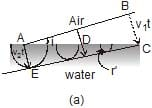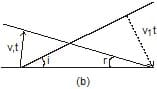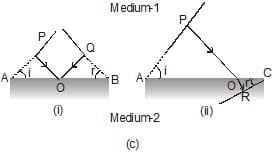• (Fig.) (a) Huygens' construction for the (a) refracted wave. (b) Reflected wave. (c) Calculation of propagation time between wavefronts in (i) reflection and (ii) refraction.
• We now calculate the total time to go from one wavefront to another along the rays. From Fig. (c), we have we have Total time for light to reach from P to Q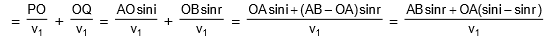• Different rays normal to the incident wavefront strike the surface at different points O and hence have different values of OA. Since the time should be the same for all the rays, the right side of equation must actually be Independent of OA.
• The condition, for this to happen is that the coefficient of OA in Eq. (should be zero, i.e., sin i = sin r. We, thus, have the law of reflection, i = r. Figure also shows refraction at a plane surface separating medium 1 (speed of light v1) from medium 2 (speed of light v2).
• The incident and refracted wavefronts are shown, making angles i and r' with the boundary. Angle r' is called the angle of refraction. Rays perpendicular to these are also drawn. As before, let us calculate the time taken to travel between the wavefronts along any ray.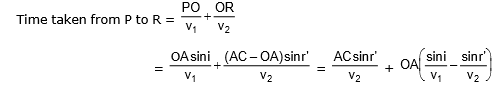• This time should again be independent of which ray we consider. The coefficient of OA in Equation is,
therefore, zero,. That is,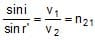where n21 is the refractive index of medium 2 with respect to medium 1. This is Snell's law of, refraction that we have already dealt with from Eq. n21 is the ratio of speed of light in the first medium (v1) to that in the second medium (v2). Equation is, known as the Snell's law of refraction. If the first medium is vacuum, we have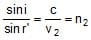where n2 is the refractive index of medium 2 with respect to vacuum, also called the absolute refractive index of the medium. A similar equation defines absolute refractive index n1 of the first medium. From Eq. we then get
n21 =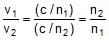• The absolute refractive index of air about 1.0003, quite close to 1. Hence, for all practical purposes, absolute refractive index of a medium may be taken with respect to air. For water, n1 = 1.33, which means v1 =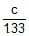, i.e. about 0.75 times the speed of light in vacuum. The measurement of the speed of light in water by Foucault (1850) confirmed this prediction of the wave theory.

Behaviour of prisms, lenses, and mirrors

Once we have the laws of reflection and refraction, the behaviour of prisms. lenses, and mirrors can be understood.  Here was just describe the behaviour of the wavefronts in these three cases (Fig)

• Consider a plane wave passing through a thin prism. Clearly, the portion of the incoming wavefront which travels through the greatest thickness of glass has been delayed the most. Since light travels more slowly in glass. This explains the tilt in the emerging wavefront.
• A concave mirror produces a similar effect. The centre of the wavefront has to travel a greater distance before and after getting reflected, when compared to the edge. This again produces a converging spherical wavefront.
• A concave mirror produces a similar effect. The centre of the wavefront has to travel a greater distance before and after getting reflected, when compared to the edge. This again produces a convering spherical wavefront.
• Concave lenses and convex mirrors can be understood from time delay arguments in a similar manner. One interesting property which is obvious from the pictures of wavefronts is that the total time taken from a point on the object to the corresponding point on the image is the same measured along any ray (Fig.). For example, when a convex lens focuses light to form a real image, it may seem that rays going through the centre are shorter. But because to the slower speed in glass, the time taken is the same as for rays travelling near the edge of the lens.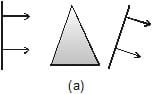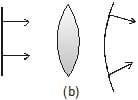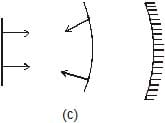Extra Portion for IIT-Mains

Diffraction due to a Single Slit

When a parallel beam of light is incident normally on a single slit, the beam is diffracted from the slit and the diffraction pattern consists of a very intense central maximum having on either sides minima, and secondary maxima alternately.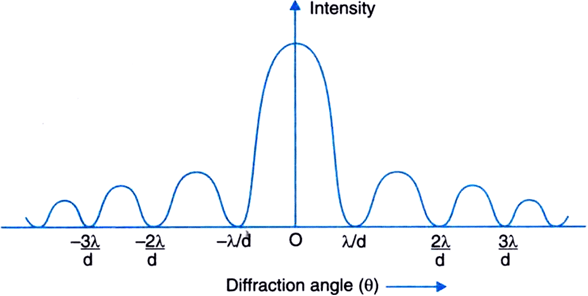If a is width of slit and q the angle of diffraction, then the directions of maxima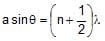n = 1, 2, 3 .............

The position of nth minima are given by

a sinθ = nl

Half-width of central maximum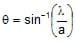Total width of central maximum,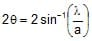Linear Width : If D is the distance of screen from slit and y is the distance of nth minima from the centre of the principal maxima, then Linear half-width of central maximum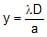Total linear width of central maximum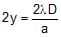Resolving Power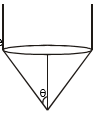1. Telescope : If a is the aperture of telescope and l the wavelength, then resolving limit of telescope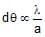For spherical aperture,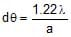2. Microscope : For microscope, q is the well resolved semi-angle of cone of light rays entering the telescope, then limit of resolution =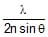where n sinθ is called numerical aperture, NA

Polarisation

The phenomenon of asymmetry of vibrations about the direction of propagation of light is called polarisation.

1. Unpolarised Light : The light having vibrations of electric field vector in all possible directions perpendicular to the direction of wave propagation is called the oridinary (or unpolarised) light.

2. Plane Polarised Light : The light having vibrations of electric field vector in only one direction perpendicular to the direction of propagation of light is called plane polarised light.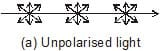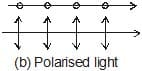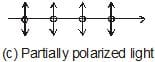3. Polarisation by Reflection : Brester's Law : If unpolarised light falls on a transparent surface of refractive index n at a certain angle lp, called polarising angle, then reflected light is plane polarised.

This is called Brewter's law. It is given by n = tan ip

Under this condition the reflected refracted rays are mutually perpendicular i.e.,

ip r = 90°

where r is angle of refraction into the plane.

4. Malus Law : It states that if polarised light is passed through an analyser, the intensity of light transmitted µ cos2q , where q is angle between planes of transmission on polariser and analyser i.e.,

I = I0 cos2q (Malus Law)

In incident light in unpolarised, then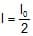,

since (cos2q)average for all directions 1/2.

5. Polaroid : Polaroid is a device to produce and detect plane polarized light.

Some Uses of Polaroids are :

(i) Sun glasses filled with polaroid sheets protect our eyes from glass.

(ii) Polaroids reduce heat light glass of motor carry being driven at light.

(iii) Polaroids are used in three-dimensional picture i.e., in holography.

7. Analysis of given light beam : For this given light beam is made incident on a polaroid (or Nicol) and the polaroid/Nicole is gradually rotated :

(i) If light beam shown no variabtion in intensity, then the given beam is umpolarised.

(ii) If light beam shows variation in intensity but the minimum intensity is non-zero, then the given beam is partially polarised.

(iii) If light beam shows variation in intensity and intensity becomes zero twice in a rotation then the given beam of light is plane polarised.

The document Reflection & Refraction of Waves - Notes | Study Physics Class 11 - NEET is a part of the NEET Course Physics Class 11.
All you need of NEET at this link: NEET

## Physics Class 11

127 videos|464 docs|210 tests
 Use Code STAYHOME200 and get INR 200 additional OFF

## Physics Class 11

127 videos|464 docs|210 tests

### How to Prepare for NEET

Read our guide to prepare for NEET which is created by Toppers & the best Teachers

Track your progress, build streaks, highlight & save important lessons and more!

,

,

,

,

,

,

,

,

,

,

,

,

,

,

,

,

,

,

,

,

,

;